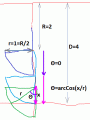# Mathematical wonders right in front of us!

#### xox

Joined Sep 8, 2017
517
Just wanted to share a fascinating little gem of maths that's been hiding in plain sight from us all along. Let's see how geometry itself is embedded in pure randomness!

The experimental setup is quite simple. All you need is something flat and grid-like (a tile floor for instance) and a thin "stick" of material cut to exactly half the width of the squares of our grid. You might want to have a pencil and paper or smartphone handy for calculations too.

Now all there is to do is toss our "stick" in the air and let it land on the grid. Be sure to keep count of the total number T of tosses. So if it lands on any of the horizontal lines of the grid then we simply add 1 to a counter C. (You could instead choose vertical line crossings by convention of course).

Repeat as many times as possible, the more the better. Once you've run a good number of trials you can begin to see the magic emerge. Divide the total T by the number of crossings C and what do you get? It should be a number very close to 3. In fact, as the number of trials increases, you should notice this ratio approach a very special number indeed: 3.1415926(...). That's right, it's Pi!

Okay so as I mentioned earlier, the accuracy of the result is strictly dependent upon the number of trials T, so the best approach here is to spread out the experiment over a number of days if possible. It can even be conducted continuously...provided one has the patience to do such a thing of course. But (filtering fluctuations aside) if you did you would get an increasingly more accurate approximation of the famous circle constant. (To be sure the ACTUAL circle constant is Tau, or twice Pi, but we won't get into that here!)

For reference: Buffon's Needle Problem.

Last edited:

#### atferrari

Joined Jan 6, 2004
4,170
For tiles 25x25 cm, what are the dimensions of the stick?

#### xox

Joined Sep 8, 2017
517
For tiles 25x25 cm, what are the dimensions of the stick?
12.5 cm long and, preferably, infinitely thin!

•atferrari

#### ci139

Joined Jul 11, 2016
1,696
the bad thing is - it takes a 3D model to do this programmatically in a 'puter - not a problem - but the time it takes
or then not - it's a 2D , where the LR UD symmetry requires only 1 4-th of the square for randomizing the stick's center ??? and then accordingly only 90° angle randomization . . . comes like max distance where the stick still can touch the horizontal . . . integrated towards the horizontal with increasing angle - that - from 0° to 90° . . . ← it does not take the vertical lines at all to get this . . .
. . . ↑ it does not take the area at all - just the stick approaching to horizontal - integrating over the "hit" angle for distance - the angle changing from vertical to horizontal . . . it does not take even the RND !
___
about https://www.symbolab.com/solver/definite-integral-calculator/\frac{1}{\frac{\pi}{2}}\int_{0}^{1}\left(arccos\left(r\right)\right)dx ← gives $$\displaystyle{\frac2{\pi}}$$ that needs to be adjusted to $$\displaystyle{\frac{0+\frac2{\pi}}2}$$
so - the squares pattern actually boosts the process by factor 2 as $$\displaystyle{\frac{N_\text{HORIZ.}+N_\text{VERT.}}{2·N}}$$ and also improves the stats. precision by the factor of 2
___
!!! no matter that it is still phenomenal that there has been presented - an easy to implement - statistical π computing method !!!
___Last edited: